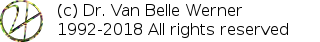Home Papers Reports Projects Code Fragments Dissertations Presentations Posters Proposals Lectures given Course notes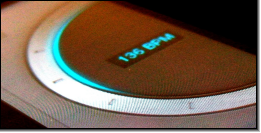# Scratching in Discontinuous Time

Werner Van Belle1 - werner@yellowcouch.org, werner.van.belle@gmail.com

1- Yellowcouch;

Abstract :  Scratching is the act of stopping a turntable and then moving the vinyl back and forth, during which the needle still captures a soundwave, leading to a slowed-down or sped-up, pitch shifted output. On physical turntables this process is natural. (It is likely that without turntables, nobody would have invented scratching in the first place, and we would never have to suffer the resulting Dj scratch cult). Digitally however, it requires some mathematics to deal with the discontinous nature of user interface events.

Keywords:  Digital Scratching, Digital DJ-ing
Reference:  Werner Van Belle; Scratching in Discontinuous Time; Signal Processing; YellowCouch; July 2011

 Introduction Deciding the speed of an acceleration change Graphics Time to reach ? Conclusion

# Introduction

A first approach to this problem consists of grabbing the current playposition when a scratch starts. Every time a new scratchevent arrives, the new required positions (around the original) is calculated and deep-setted into the player. This is a disaster: it leads to jumps and ticks in the produced audiostream.

To smother out this problem, it is possible to measure the required position-change and map that to the playing speed. In this case we never really change the position directly, we merely affect it through the velocity. The drawback of this method is that, with every new event, the pitch of the next block is altered. This doesn't sound very well because the pitch changes can be quite large. The second problem with this technique is that it becomes difficult to have a 'stable' position. A sweep up and down along the scratchpad will not necessarily lead back to the same position.

An easy fix to solve the pitch change problem is to gradually modify the velocity: v=v+0.1(v_{t}-v), with v_{t} being the target velocity. This approach is flawed due to an inherent contradiction: either we have a slow pickup with small pitch/velocity changes or we have a fast pickup but with strong pitch/velocity changes. A slow pickup is bad because a sweep across the scratchpad will never sufficiently change the playposition.

Another approach one might consider is trying to analyze the semantics of a scratch. Instead of actually calculating mouse events, just the local extrema of the scratches are kept. This stabilizes the velocity but still doesn't sound good because the we must wait for multiple events to occur.)

# Deciding the speed of an acceleration change

Because setting the position didn't work, and because the first differential improved the results, but still suffered from strong pitch changes, we now continue directly to the third differential.

We would like to modify the acceleration slowly, such that we, starting at a specific velocity reach a target position in a given time. ... That means that we are looking for an equation of the form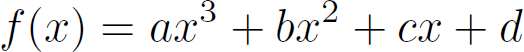in which x is the realtime (how much samples we produced) and f(x) is the position from where the sample is to be retrieved. We always assume that our current position is at (0,0), which means that f(0)=0, which implies that d must be 0.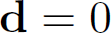When a scratchevent comes in we notify the player that a certain position must be reached at a specific time in the future. The retrieval position to reach will be called d_{y}. The time we have to reach it is called d_{x}. Hence: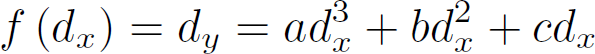To get a better grip on this equation (and solve it), we must consider the requirements on the velocity. At position 0 we have an initial velocity of v_{0}.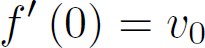At time d_{x} our velocity should be 0, so that in case the new event was a 'stopscratch', we won't overshoot the target.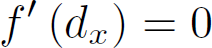Let's now look at the differential of f, so that we can deal with these two requirements.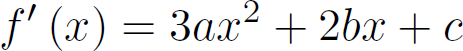Combining this differential and the initial velocity equation gives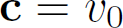which leaves us two equations in two unknowns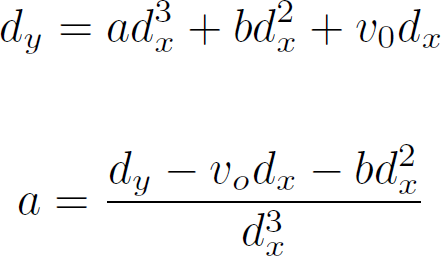Based on the first differential and the final velocity requirement, we get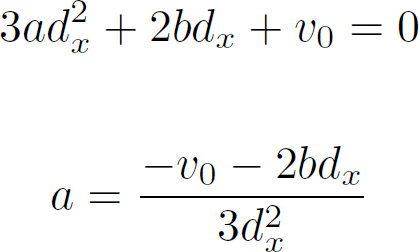Combining these two equations through the acceleration a gives: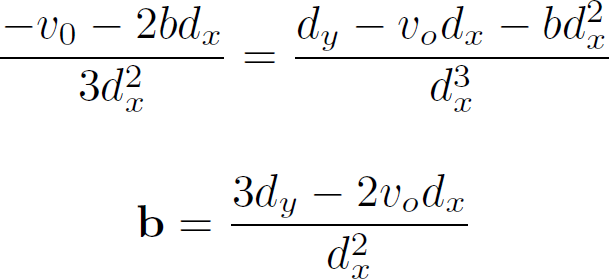Which when inserting b back into either of them gives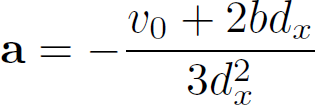# Graphics

The red line in the following graphics illustrates what happens if we specify that we want to reach within 40000 samples, retrieval position 1000. The three graphics show, position, velocity and acceleration. As wanted, the position changes very smoothly. The velocity changes quadratically, and thus without discontinuities, and the acceleration linearly. In the beginning the 'scratch' accelerates up to half of the allotted time, after which it slows decelerates again.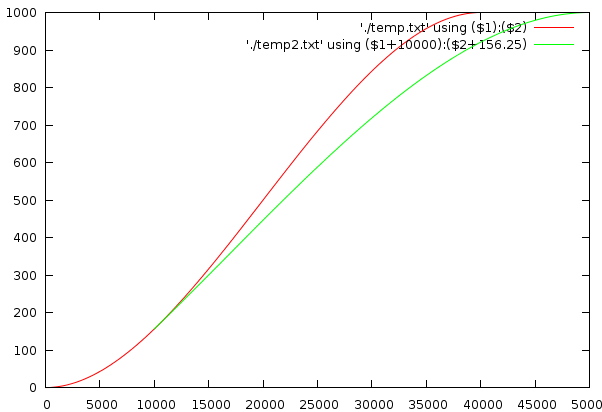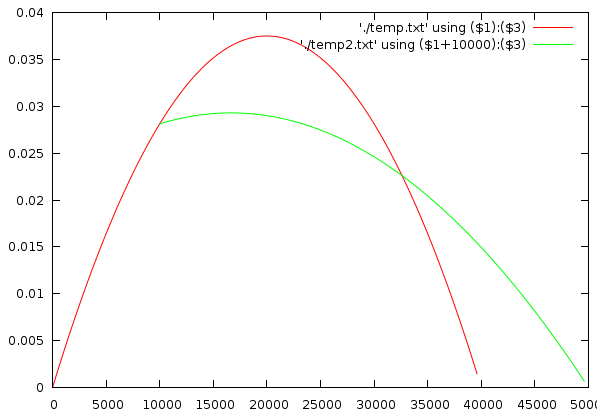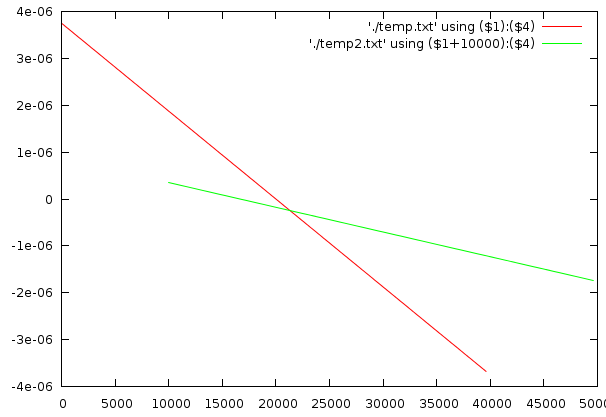Time x Position Time x Velocity Time x Acceleration

Figure 1: In each of these 3 graphs, playing time is set horizontally. Vertically, the sample retrieval position, its velocity and acceleration are respectively plotted. The red line show how a target at (40000,1000) will be approached. The green line illustrates what happens if, at position 10000, we suddenly need to reach (50000,1000).

What happens when a new scratch event comes in ? In that case the target time and target position are recalculated and the old equation is simply forgotten. Now we verify that the old curve and the new curve matches up properly. That means: no strong velocity changes and continuing at the same position as before. Intuitively, the equation is created such that between the origin and the target position a relatively stable velocity is achieved. This makes it possible to superimpose two graphics around the center, with their positions only slightly differing.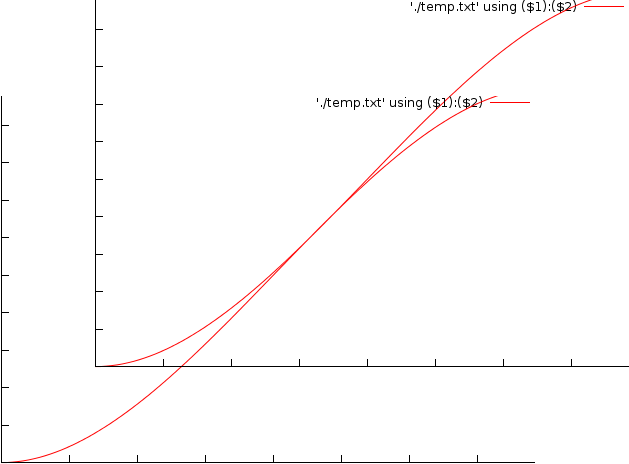When a new scrath event comes in we must recalculate the targetposition and acceleration change. This graph illustrates how two position curves might match if the required change is not too drastic. See text for more.

To explain the above in more detail. Assume that we have a starting point at 0,0 and a target position of (40000,1000). After 10000 samples we obtain a scratchevent that specifies that the target position now is (50000,1000). In figure 1, the red line is the approach to (40000,1000). The green line is the recalculated position-path, in which we see how the new curve starts out where it was at that particular position in time. In the velocity plot we see that the velocity also starts out where it was at that time. Aside from the discontinuity, it remains relatively flat; which is normal since the target retrieval position didn't change much. We only obtained more time to reach it, so the algorithm doesn't need to accelerate further to reach it. In the acceleration plot we see that the acceleration is changed fairly strongly. But this is already the 3th differential: the change in acceleration. Or to confuse people: the 4th differential is a constant.

# Time to reach ?

What are good values for dx and dy ? Preferably, between the creation of a positioning curve and the creation of the next curve, we would like to have the largest overlap as possible. It appears that this occurs in the middle of the first curve. This means that we would prefer to receive a new event at dx/2. If the events are handled at a period of around 150 milliseconds, then a dx value of 300 milliseconds would be appropriate to have a smooth scratching going on.

# Conclusion

The above equations create a position-path for the purpose of scratching, in which both 'potential futures' are met. Each path will reach the required target and will pick up speed relatively fast, and if no new scratch event comes in will come to a halt. As soon as new 'scratchevent' comes in the curve can be calculated and will smoothly pick up were the old one left off. The equation is so constructed that strong pitch changes will not occur. This has been achieved by modifying the acceleration linearly.

 http://werner.yellowcouch.org/werner@yellowcouch.org